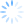# 慢羊羊的空间

``````///////////////////////////////////////////////////
// 程序名称：基于 DDA 算法画任意斜率的直线
// 编译环境：Visual C++ 6.0 / 2010，EasyX 2011惊蛰版
// 作　　者：yangw80 <yw80@qq.com>
// 最后修改：2011-4-26
//
#include <graphics.h>
#include <conio.h>

// 四舍五入
int Round(float x)
{
return (int)(x < 0 ? x - 0.5 : x + 0.5);
}

// 使用 DDA 算法画任意斜率的直线（包括起始点，不包括终止点）
void Line_DDA(int x1, int y1, int x2, int y2, int color)
{
float x, y;		// 当前坐标点
float cx, cy;	// x、y 方向上的增量

int steps = abs(x2 - x1) > abs(y2 - y1) ? abs(x2 - x1) : abs(y2 - y1);

x = (float)x1;
y = (float)y1;
cx = (float)(x2 - x1) / steps;
cy = (float)(y2 - y1) / steps;

for(int i = 0; i < steps; i++)
{
putpixel(Round(x), Round(y), color);	// 在坐标 (x, y) 处画一个 color 颜色的点
x += cx;
y += cy;
}
}

// 主函数
void main()
{
initgraph(640, 480);

// 测试画线
Line_DDA(100, 1, 1, 478, GREEN);
Line_DDA(1, 478, 638, 1, GREEN);

// 按任意键退出
getch();
closegraph();
}``````